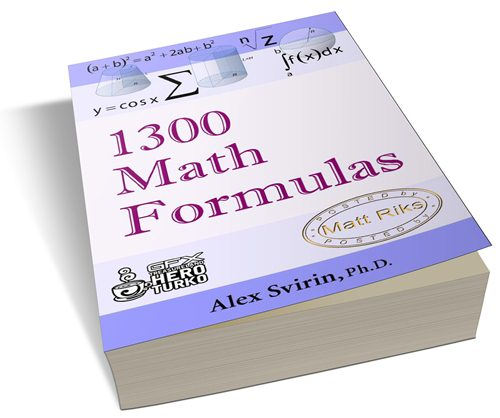Refer a friend and get % off! They'll get % off too.1300 mathematic formulas

By Rajib Kumar

This is an e-book which contains 1300 formulas.

CONTENTS:-

NUMBER SETS
ALGEBRA
GEOMETRY
TRIGONOMETRY
MATRICES AND DETERMINANTS
VECTORS
ANALYTIC GEOMETRY
DIFFERENTIAL CALCULUS
INTEGRAL CALCULUS
DIFFERENTIAL EQUATIONS
SERIES
PROBABILITY